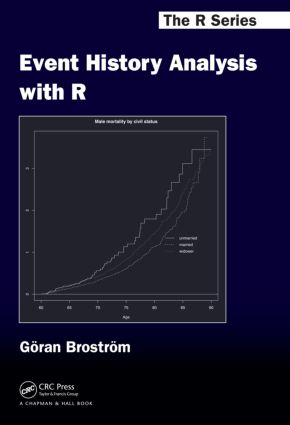Event History Analysis with R

1st Edition

CRC Press

236 pages | 75 B/W Illus.

Purchasing Options:\$ = USD
Hardback: 9781439831649
pub: 2012-04-03
SAVE ~\$19.19
\$95.95
\$76.76
x
eBook (VitalSource) : 9781315373942
pub: 2018-09-03
from \$47.98

FREE Standard Shipping!

Description

With an emphasis on social science applications, Event History Analysis with R presents an introduction to survival and event history analysis using real-life examples. Keeping mathematical details to a minimum, the book covers key topics, including both discrete and continuous time data, parametric proportional hazards, and accelerated failure times.

Features

• Introduces parametric proportional hazards models with baseline distributions like the Weibull, Gompertz, Lognormal, and Piecewise constant hazard distributions, in addition to traditional Cox regression
• Presents mathematical details as well as technical material in an appendix
• Includes real examples with applications in demography, econometrics, and epidemiology
• Provides a dedicated R package, eha, containing special treatments, including making cuts in the Lexis diagram, creating communal covariates, and creating period statistics

A much-needed primer, Event History Analysis with R is a didactically excellent resource for students and practitioners of applied event history and survival analysis.

Reviews

"This book in The R Series from Chapman & Hall acts much as a companion to the R package eha by the same author. … If one wants to analyse such data using R, then the book is well worthwhile. Although it is written more from the point of view of a reader comfortable in using R [and] wanting to learn more about demographic data, it also offers something for the demographer looking to extend the scope of their analyses. … the depth of treatment is about right to form the core of a lecture course …"

—Mark Bebbington, Australian & New Zealand Journal of Statistics, 2013

Preface

Event History and Survival Data

Introduction

Survival Data

Right Censoring

Left Truncation

Time Scales

Event History Data

More Data Sets

Single Sample Data

Introduction

Continuous Time Model Descriptions

Discrete Time Models

Nonparametric Estimators

Doing it in R

Cox Regression

Introduction

Proportional Hazards

The Log-Rank Test

Proportional Hazards in Continuous Time

Estimation of the Baseline Hazard

Explanatory Variables

Interactions

Interpretation of Parameter Estimates

Proportional Hazards in Discrete Time

Model Selection

Male Mortality

Poisson Regression

Introduction

The Poisson Distribution

The Connection to Cox Regression

The Connection to the Piecewise Constant Hazards Model

More on Cox Regression

Introduction

Time-Varying Covariates

Communal covariates

Tied Event Times

Stratification

Sampling of Risk Sets

Residuals

Checking Model Assumptions

Fixed Study Period Survival

Left- or Right-Censored Data

Parametric Models

Introduction

Proportional Hazards Models

Accelerated Failure Time Models

Proportional Hazards or AFT Model?

Discrete Time Models

Multivariate Survival Models

Introduction

Frailty Models

Parametric Frailty Models

Stratification

Competing Risks Models

Introduction

Some Mathematics

Estimation

Meaningful Probabilities

Regression

R Code for Competing Risks

Causality and Matching

Introduction

Philosophical Aspects of Causality

Causal Inference

Aalen’s Additive Hazards Model

Dynamic Path Analysis

Matching

Conclusion

Basic Statistical Concepts

Introduction

Statistical Inference

Asymptotic theory

Model Selection

Survival Distributions

Introduction

Relevant Distributions in R

Parametric Proportional Hazards and Accelerated Failure Time Models

A Brief Introduction to R

R in General

Some Standard R Functions

Writing Functions

Graphics

Probability Functions

Help in R

Functions in eha and survival

Reading Data into R

Survival Packages in R

Introduction

eha

survival

Other Packages

Bibliography

Index